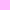Go to Home of Graduate School of Math. Sci.
Introduction of Yoshikazu GIGATopIntroductionPublication
ListSeminars/
Conferences
 Affiliation: Graduate School of Mathematical Sciences, University of Tokyo Research Field: Nonlinear Analysis Research Subject: Mathematical Analysis on Differential Equations describing Nonlinear Diffusive Phenomena Current Research: Various differential equations have been proposed with the purpose to model phenomena in nature such as motion of fluid, crystal growth and to handle problems in technology for example in image processing. My major research topic is nonlinear diffusion equations which form an important class of partial differential equations. ( A partial differential equation is a differential equation having several independent variables.) My particular interest lies in solvability of partial differential equations under given conditions and in tracking behavior of solutions in a rigorous mathematical framework. Keywords: Navier-Stokes equations, surface evolution equations, blow-up phenomena of solutions, viscosity solutions, variational analysis, nonlinear diffusion equations Awards: Nissan Science Award (Nissan Science Foundation) (1997) Highly Cited Researchers (ISI Thomson Scientific) (2002) Autumn Award (Mathematical Society of Japan) (1999) Inoue Prize for Science (Inoue Foundation for Science) (2004) Medal of Honour with Purple Ribbon (2010) Fellow of American Mathematical Society (2012) Fellow of Japan Society for Industrial and Applied Mathematics (2014)
Last updated: October 14, 2018
Copyright 2018 Yoshikazu Giga. All Rights Reserved.